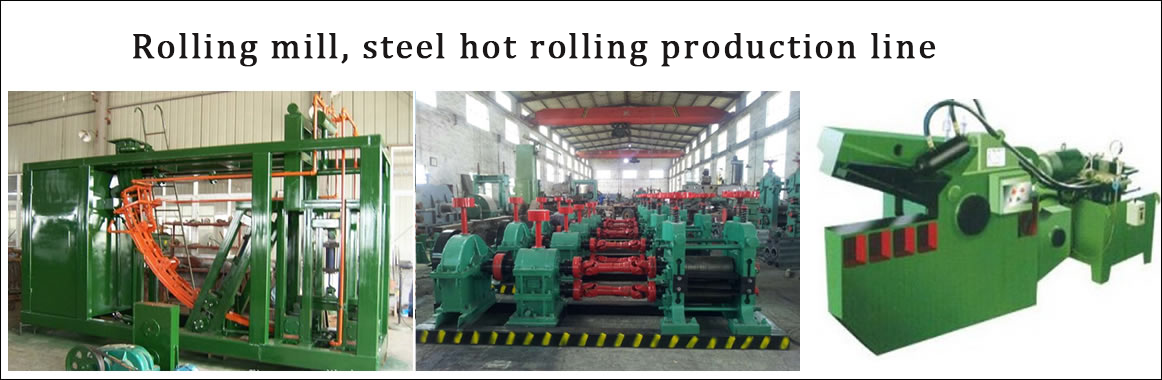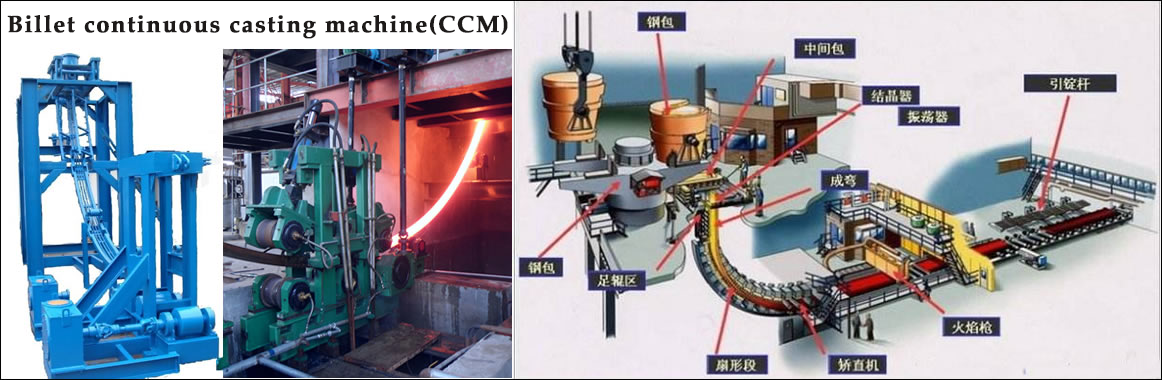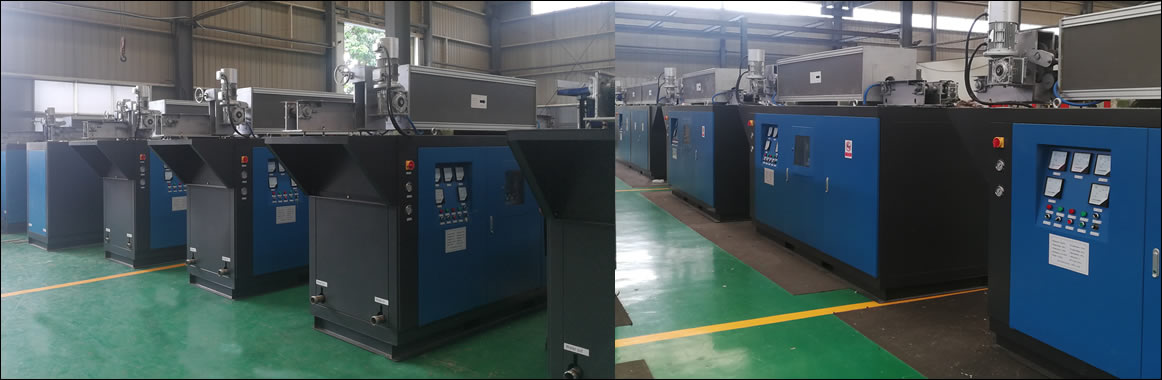••••••Contact
Tel: 18037961302
Sales hot line ( 24 hours  service): 18037961302

E-Mail: firstfurnace@gmail.com

whatsapp:+8618037961302

Adress: Luoxin Industrial Park, Luoyang, Henan
Electric furnace principle（原理）

Thyristor selection parameters for 1000kw induction melting furnace

The designed incoming line voltage is 380V, and the following data can be obtained by calculation:

DC voltage Ud=1.35×380V=510V

DC current Id=1000000÷510=1960A

Intermediate frequency voltage Us=1.5×Ud =765V

Rated silicon rectifier current IKP=0.38×Id=745A

Rated silicon rectifier voltage UV=1.414×UL=1.414×510V=721V

Inverter silicon rated current Ikk=0.45×19600=882A

Inverter silicon rated voltage UV=1.414×Us=1082V

SCR model selection scheme: choose Xiangfan Taiji SCR:

Because it adopts 6-pulse single rectifier output, the rectifier SCR selects KP2000A/1400V (a total of 6), that is, the rated current is 2000A and the rated voltage is 1400V. Compared with the theoretical value, the voltage margin is 1.94 times and the current margin is 2.68 times.

The inverter thyristor selects KK2500A/1600V (four in total), that is, the rated current is 2500A, and the rated voltage is 1600V. Compared with the theoretical value, the voltage margin is 1.48 times, and the current margin is 2.83 times.